• 267KB qq_35524157 2018-01-13 23:00:04
•5星
1KB xixi415415 2012-09-01 07:46:38
•4星
85KB mostovoi1234 2011-01-20 07:16:17
• 循环赛日程表分治递归求解 循环赛日程表 分治 递归

分治策略，将所有的选手分为两半，n个选手的比赛日程表就可以通过为n/2个选手设计的比赛日程表来决定。递归地用对选手进行分割，直到只剩下2个选手时，比赛日程表的制定就变得很简单。这时只要让这2个选手进行比赛...

设计一个满足以下要求的比赛日程表：

(1)每个选手必须与其他n-1个选手各赛一次；
(2)每个选手一天只能赛一次；
(3)循环赛一共进行n-1天。

按分治策略，将所有的选手分为两半，n个选手的比赛日程表就可以通过为n/2个选手设计的比赛日程表来决定。递归地用对选手进行分割，直到只剩下2个选手时，比赛日程表的制定就变得很简单。这时只要让这2个选手进行比赛就可以了。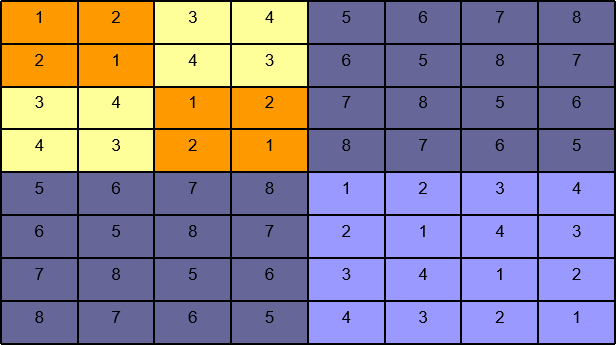如上图，所列出的正方形表是8个选手的比赛日程表。其中左上角与左下角的两小块分别为选手1至选手4和选手5至选手8前3天的比赛日程。据此，将左上角小块中的所有数字按其相对位置抄到右下角，又将左下角小块中的所有数字按其相对位置抄到右上角，这样我们就分别安排好了选手1至选手4和选手5至选手8在后4天的比赛日程。依此思想容易将这个比赛日程表推广到具有任意多个选手的情形。

问题求解

(1)用一个for循环输出日程表的第一行 for(int i=1;i<=N;i++) a[i] = 1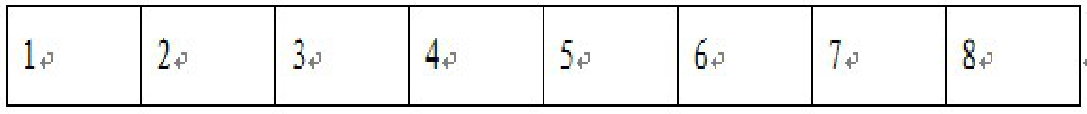(2)然后定义一个m值，m初始化为1，m用来控制每一次填充表格时i（i表示行）和j（j表示列）的起始填充位置。

(3)用一个for循环将问题分成几部分，对于k=3，n=8，将问题分成3大部分，
第一部分为，根据已经填充的第一行，填写第二行，
第二部分为，根据已经填充好的第一部分，填写第三四行，
第三部分为，根据已经填充好的前四行，填写最后四行。
可以用for (ints=1;s<=k;s++) N/=2; 描述这个过程

(4)用一个for循环对③中提到的每一部分进行划分for(int t=1;t<=N;t++)对于第一部分，将其划分为四个小的单元，即对第二行进行如下划分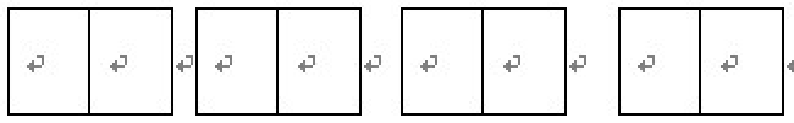同理，对第二部分（即三四行），划分为两部分，第三部分同理。

(5)最后，根据以上for循环对整体的划分和分治法的思想，进行每一个单元格的填充。填充原则是：对角线填充

for(int i=m+1;i<=2*m;i++) //i控制行
for(int j=m+1;j<=2*m;j++)  //j控制列
{
a[i][j+(t-1)*m*2]= a[i-m][j+(t-1)*m*2-m];
/*右下角的值等于左上角的值 */
a[i][j+(t-1)*m*2-m] =a[i-m][j+(t-1)*m*2];
/*左下角的值等于右上角的值 */
}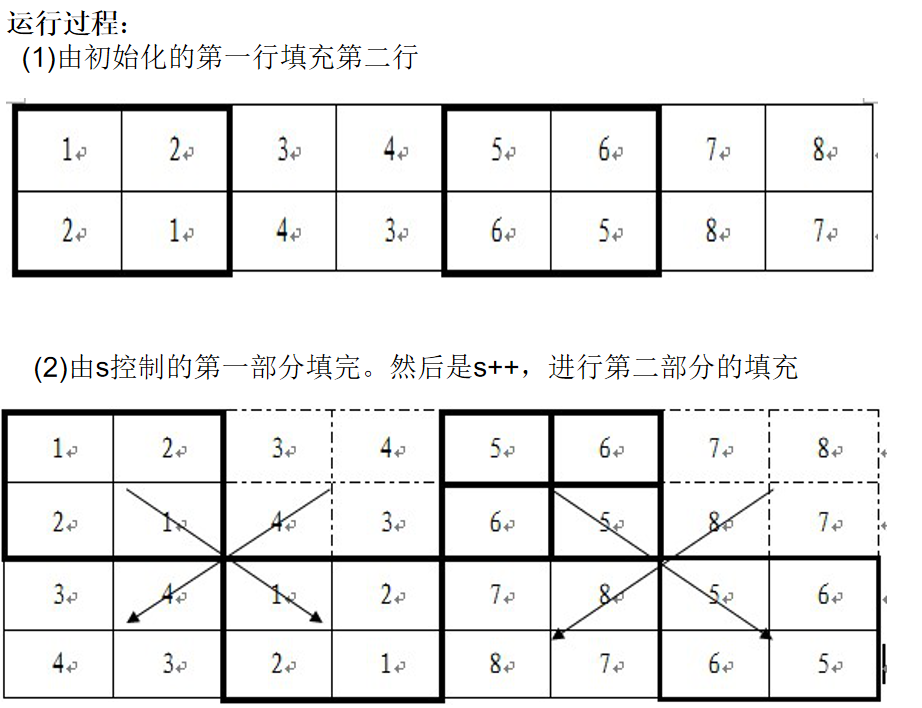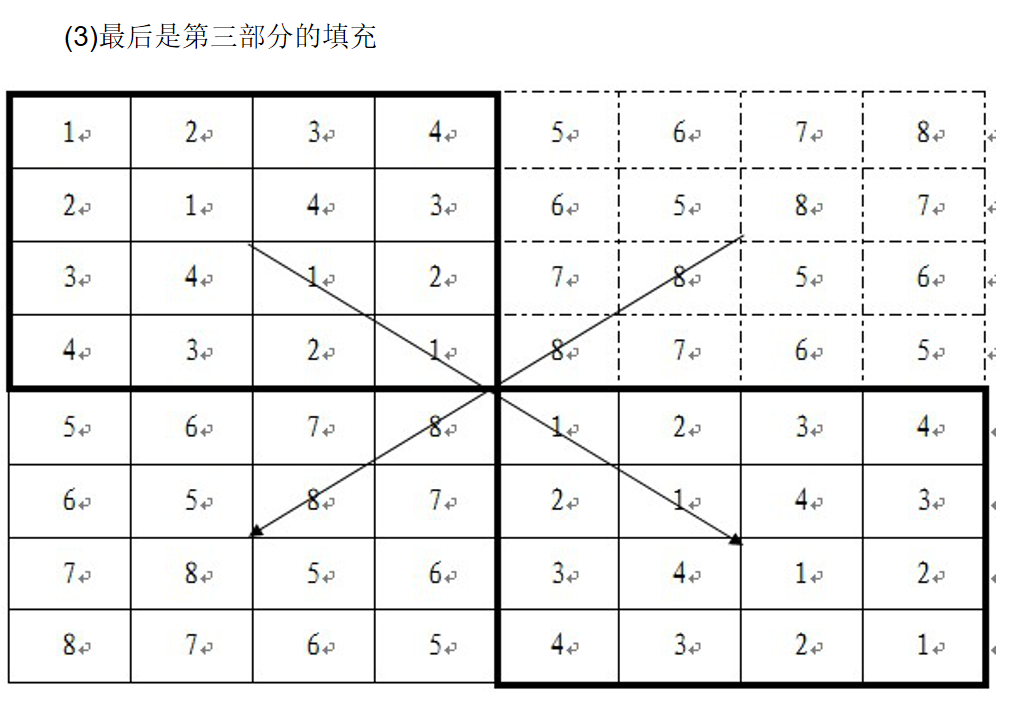算法实现

int a;
void cycle_table(int k)
{
int n=1;//共有n个运动员
for(int i=1;i<=k;i++) n=n*2;
//先填写第一行
for(int i=1;i<=n;i++) a[i]=i;
int m=1;
for(int s=1;s<=k;s++)
{
n=n/2;//每次循环的次数
for(int t=1;t<=n;t++)
for(int i=m+1;i<=2*m;i++)
for(int j=m+1;j<=2*m;j++)
{
a[i][j+(t-1)*2*m]=a[i-m][j+(t-1)*2*m-m];
a[i][j+(t-1)*2*m-m]=a[i-m][j+(t-1)*2*m];
}
m=m*2;
}

}

输入输出

输入

输入只有一行，输入一个数为k，运动员人数N=2k

输出

输出有N行N列，每个数字中间有一个空格
第一列表示每个运动员的序号，第2-N列表示N-1天的赛事安排

样例输入

3

样例输出

1 2 3 4 5 6 7 8
2 1 4 3 6 5 8 7
3 4 1 2 7 8 5 6
4 3 2 1 8 7 6 5
5 6 7 8 1 2 3 4
6 5 8 7 2 1 4 3
7 8 5 6 3 4 1 2
8 7 6 5 4 3 2 1

#include <iostream>
#include<stdio.h>
#include<stdlib.h>
using namespace std;
int a;
void cycle_table(int k)
{
int n=1;//共有n个运动员
for(int i=1;i<=k;i++) n=n*2;
//先填写第一行
for(int i=1;i<=n;i++) a[i]=i;
int m=1;
for(int s=1;s<=k;s++)
{
n=n/2;//每次循环的次数
for(int t=1;t<=n;t++)
for(int i=m+1;i<=2*m;i++)
for(int j=m+1;j<=2*m;j++)
{
a[i][j+(t-1)*2*m]=a[i-m][j+(t-1)*2*m-m];
a[i][j+(t-1)*2*m-m]=a[i-m][j+(t-1)*2*m];
}
m=m*2;
}

}
int main()
{
int k;
cin>>k;
int n=1;
for(int i=1;i<=k;i++) n=n*2;
cycle_table(k);
for(int i=1;i<=n;i++){
for(int j=1;j<=n;j++)
cout<<a[i][j]<<" ";
cout<<endl;
}
return 0;
}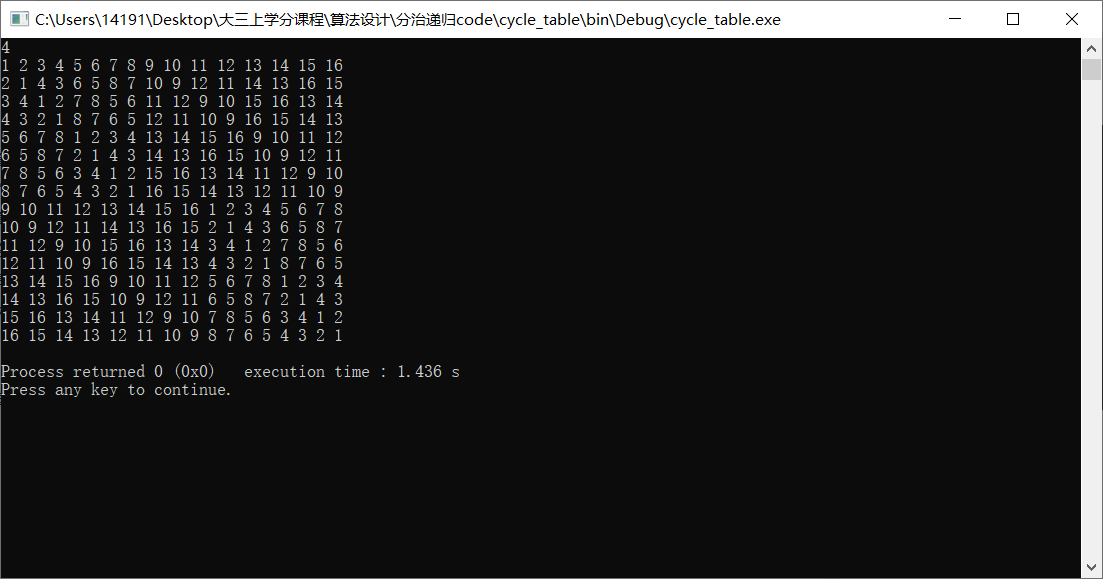展开全文practical_sharp 2019-10-05 10:16:36
• 循环赛日程表算法——分治法 算法

设有n=2^{k}个选手要进行网球循环赛，要求设计一个满足以下要求的比赛日程表： （1）个选手必须与其他n-1个选手各赛一次； （2）每个选手一天只能赛一次。 按此要求，可将比赛日程表设计成一个 n 行n-1列的二维表，...

一、问题描述：

设有n=2^{k}个选手要进行网球循环赛，要求设计一个满足以下要求的比赛日程表：

（1）个选手必须与其他n-1个选手各赛一次；

（2）每个选手一天只能赛一次。

按此要求，可将比赛日程表设计成一个 n 行n-1列的二维表，其中，第 i 行第 j 列表示和第 i 个选手在第 j 天比赛的选手。二、问题分析：

按照分治的策略，可将所有参赛的选手分为两部分，n＝2k个选手的比赛日程表就可以通过为\frac{n}{2}=2k-1个选手设计的比赛日程表来决定。递归地执行这种分割，直到只剩下2个选手时，比赛日程表的制定就变得很简单：只要让这2个选手进行比赛就可以了。

这种解法是把求解2^{k} 个选手比赛日程问题划分成依次求解2{1}、2{2}、…、2{k}个选手的比赛日程问题，换言之，2{k}个选手的比赛日程是在2^{k-1}个选手的比赛日程的基础上通过迭代的方法求得的。在每次迭代中，将问题划分为4部分：

（1）左上角：左上角为2^{k-1}个选手在前半程的比赛日程；

（2）左下角：左下角为另2{k-1}个选手在前半程的比赛日程，由左上角加2{k-1}得到，例如2{2}个选手比赛，左下角由左上角直接加2得到，2{3}个选手比赛，左下角由左上角直接加4得到；

（3）右上角：将左下角直接抄到右上角得到另2^{k-1}个选手在后半程的比赛日程；

（4）右下角：将左上角直接抄到右下角得到2^{k-1}个选手在后半程的比赛日程；

算法设计的关键在于寻找这4部分元素之间的对应关系。

#include <stdio.h>
#define MAX 100
int a[MAX][MAX];
void Copy(int tox,int toy,int fromx,intfromy,int r)
{
for(inti=0;i<r;i++)
for(intj=0;j<r;j++)
a[tox+i][toy+j]=a[fromx+i][fromy+j];
}
//构造循环赛日程表，选手的数量n=2^k
void Table(int k)
{
inti,r;
intn=1<<k;
//构造正方形表格的第一行数据
for(i=0;i<n;i++)
a[i]=i+1;
//采用分治算法，构造整个循环赛程
for(r=1;r<n;r<<=1)
for(i=0;i<n;i+=2*r)
{
Copy(r,r+i,0,i,r);
Copy(r,i,0,r+i,r);
}
printf("参赛人数为:%d\n(第i行第j列表示和第i个选手在第j天比赛的选手序号)\n",n);
for(i=0;i<=n-1;i++)
for(r=0;r<=n-1;r++)
{
printf("%d ",a[i][r]);
if(r==n-1)
printf("\n");
}
}

intmain() {
int i,r,k;
int n=2^k;
printf("比赛选手个数为n(n=2^k)，请输入参数K(K>0):\n");
scanf("%d",&k);
if(k!=0)
Table(k);
return 0;
}

展开全文qq_43633063 2020-04-15 15:59:55
•3星
202KB fcwr_zhuxin_fcwr 2011-05-22 23:23:04
• 现在要设计一个满足以下要求的比赛日程表，每个选手必须与其他n-1个选手各赛一场，每个选手一天只能赛一次，循环赛一共进行n-1天，将比赛日程表设计成n行n列，表中除了第一列，其他n-1列才是我们要的，数组下标行列...

设有n=2^k个运动员，要进行网球循环赛。现在要设计一个满足以下要求的比赛日程表，每个选手必须与其他n-1个选手各赛一场，每个选手一天只能赛一次，循环赛一共进行n-1天，将比赛日程表设计成n行n列，表中除了第一列，其他n-1列才是我们要的，数组下标行列都从0开始，第i行j列代表第（i+1）位选手在第j天的对手：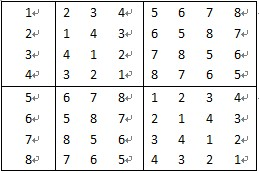题解：首先我们找到规律，此图左上角与右下角相同，右上角与左下角相同，我们可以先求出上半部分，才复制到下半部分区域，得出所求表格。
首先，初始化第一行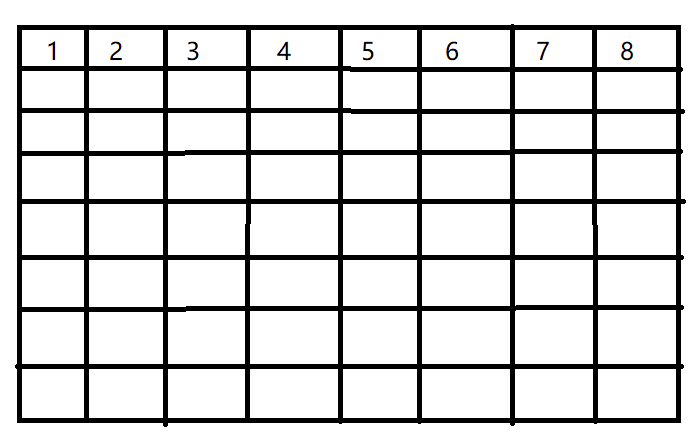从1-2开始入手，直接引用上面规律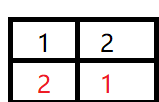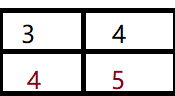扩充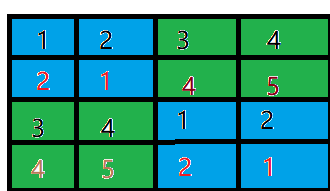求出5-8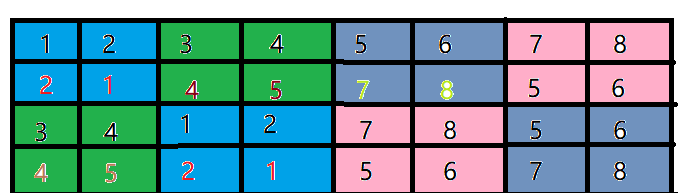求出全部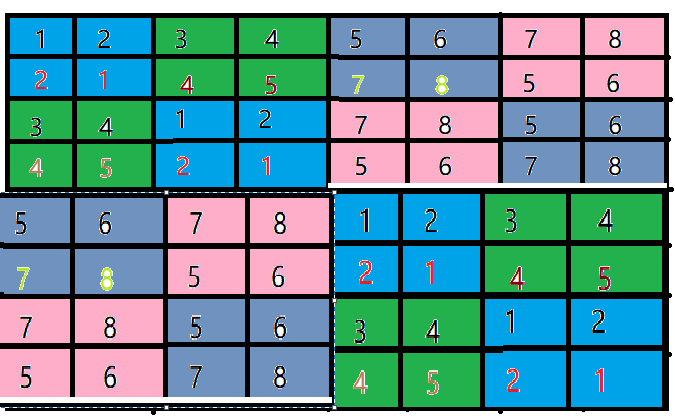代码如下所示

`cpp
#include<stdio.h>
#include<math.h>
#define N 50
int a[N][N];
void Table(int k);
void print(int k);         //输出二维数组
main()
{
int k;
printf("请输入k 的值：");
scanf("%d",&k);
Table(k);
print(k);
}
void Table(int k)//数组下标从1开始
{
int i,j,s,t;
int n=1;
for(i=1;i<=k;i++)
n*=2;                       //求总人数n
for(i=1;i<=n;i++)
a[i]=i;                  //初始化第一行排

int m=1;                          //用来控制每一次填表时i行j列的起始填充位置
for(s=1;s<=k;s++)                 //s指对称赋值的总循环次数，即分成几大步进行制作日程表
{
n=n/2;
for(t=1;t<=n;t++)              //t指明内部对称赋值的循环次数
for(i=m+1;i<=2*m;i++)
for(j=m+1;j<=2*m;j++)
{
a[i][j+(t-1)*m*2]=a[i-m][j+(t-1)*m*2-m];       //右上角等于左上角的值
a[i][j+(t-1)*m*2-m]=a[i-m][j+(t-1)*m*2];       //左下角等于右上角的值
}
m*=2;
}

}
void print(int k)
{
int i,j;
int num=pow(2,k);
for(i=1;i<=num;i++)
{
for(j=1;j<=num;j++)
{
printf("%d\t",a[i][j]);
}
printf("\n");
}
}

展开全文lixindayup 2019-10-16 15:01:06
• 分治法解决循环赛日程表 分治法 循环赛日程表

分治法解决循环赛日程表 问题描述 设有n=2^k个运动员要进行羽毛球循环赛，现要设计一个满足以下要求的比赛日程表： (1)每个选手必须与其他n-1个选手各赛一次。 (2)每个选手一天只能比赛一次。 (3)循环赛一共...

分治法解决循环赛日程表

问题描述

设有n=2^k个运动员要进行羽毛球循环赛，现要设计一个满足以下要求的比赛日程表：
(1)每个选手必须与其他n-1个选手各赛一次。
(2)每个选手一天只能比赛一次。
(3)循环赛一共需要进行n-1天。
由于n=2^k，显然n为偶数。

分治法求解思路

按分治策略，将所有的选手分为两半，n个选手的比赛日程表就可以通过为n/2个选手设计的比赛日程表来决定。递归地用对选手进行分割，直到只剩下2个选手时，比赛日程表的制定就变得很简单。这时只要让这2个选手进行比赛就可以了。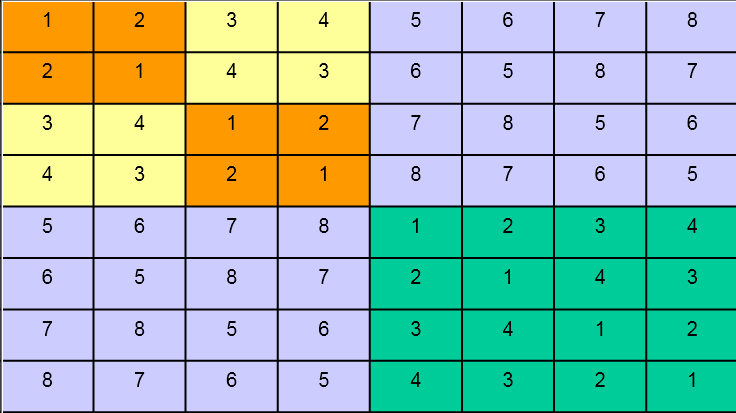(1)n=2^1个选手的比赛日程表的制定。、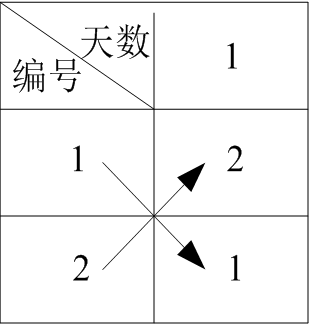(2)n=2^2个选手的比赛日程表的制定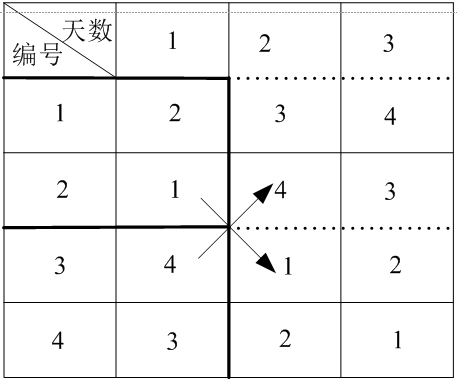(3)2^3个选手的比赛日程表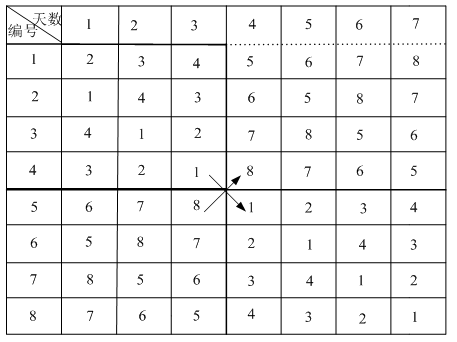……
……

算法描述

void Round_Robin_Calendar(int k,int n,int **a)
{
int i,j,s;
for(i=1; i<=n; i++)
a[i]=i;//用一个for循环输出日程表的第一行

int m = 1;//m用来控制每一次填充数组时i(i表示行)和j(j表示列)的起始填充位置
for(s=1; s<=k; s++)//将问题划分为k部分，依次处理
{
n/=2;
for(int t=1; t<=n; t++)//每一部分的问题进行单元格的填充
for(i= m+1; i<=2*m; i++)//控制行
for(int j=m+1; j<=2*m; j++)//控制列
{
a[i][j+(t-1)*m*2] = a[i-m][j+(t-1)*m*2-m];
a[i][j+(t-1)*m*2-m] = a[i-m][j+(t-1)*m*2];
}
m *= 2;
}
}

结果演示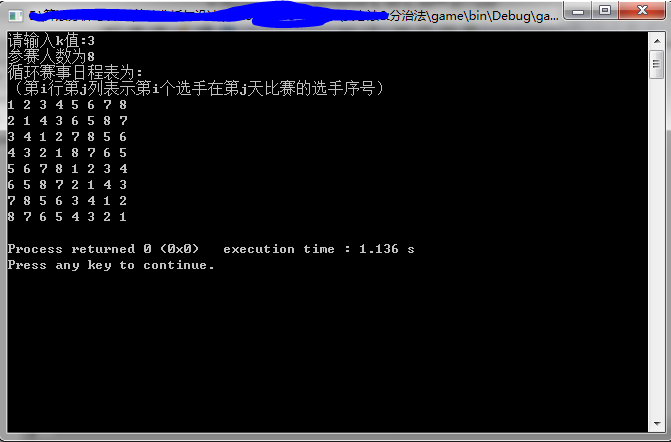工程下载地址分治法解决循环赛日程表

展开全文qq_35524157 2018-01-13 23:12:26
• 分治法求循环赛日程表 分治法 循环赛日程表 递归

ColourfulTiger 2018-05-29 16:07:33
• 分治法之循环赛日程表的理解和解题思路 算法 分治法 循环日程赛 算法思路

qq_32919451 2018-06-09 18:05:17
• weixin_39809584 2020-12-15 19:01:08
• 4KB weixin_44425171 2019-05-31 23:41:59
• 分治法--循环赛日程表 java 算法 分治算法

weixin_44721537 2020-02-01 20:36:53
• 用分治法解决循环赛日程表 递归 分治

conquern 2017-11-16 09:15:35
• 循环赛日程表，分治法（n为任意数，n=2^k），多边形轮转法（n为任意数），递归和指针，共五种解决方案。 循环赛日程表 分治法 多边形轮转法

m0_37872090 2018-04-27 09:43:17
• weixin_35286137 2021-05-24 10:46:53
• 分治法解决循环赛事日程表（非常详细！）图文并茂-Java代码实现 分治法 循环赛日程安排

BigData_Mining 2019-11-13 14:45:45
• qq_27437197 2019-01-07 22:56:28
• qq_43534524 2020-04-22 21:22:15
• weixin_39534780 2021-02-10 08:39:22
• zhuge2017302307 2018-12-29 20:11:49
• 分治法实现循环赛日程安排(递归和非递归) c++ 分治算法 算法

weixin_43589404 2020-04-23 17:04:11
• weixin_30868807 2021-05-23 12:57:43
• weixin_33780516 2021-03-14 14:37:51
• wly_2014 2016-05-12 23:00:13
• 循环赛日程表-分治算法 C语言 循环赛5星
5KB u013929477 2014-10-21 10:02:23...